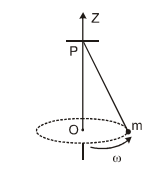# Rotational motion problems with solutions

Question -1
Find the Moment of Inertia of a sphere with axis tangent to it?
Solution
The moment of inertia of the sphere about the axis passing through the center us
$I_C=\frac {2}{5}MR^2$
Using Parallel axis theorem, Moment of inertia through the tangent is given by
$I_T =I_C + MR^2$
or
$I_T= {7}{5}MR^2$

Question -2
A car accelerates uniformly from rest and reaches a Speed of 22.0 m/s in 9.00 s. If the radius of a tire is 29 cm, find the number of revolutions the tire makes during this motion, assuming that no slipping occurs.
Solution
From $v=u+at$
$a= \frac {v}{t}$
Now Distance covered
$s= \frac {1}{2}at^2 = \frac{1}{2}v t$
Number of revolutions= $\frac {s}{2 \pi r}$
Substituting the values
Number of revolutions=54.3 revolutions

Question 3
A cylinder of Radius 20 cm is rolling along the surface with constant speed of 80 cm/sec
a. What is the rotational speed of the cylinder about its axis?
b. What is the magnitude and direction of the acceleration of the points on it surface.
c. what is the velocity of the point at the top of the cylinder at any moment
d. what is the velocity of the point of the cylinder which is in contact with the floor at any moment
Solution
a. Rotational speed $\omega = \frac {v}{R} = \frac {80}{20} = 4 rad/s$
b.Since there is no acceleration for cylinder , $\alpha = 0$ and we have just radial acceleration
$a_r= \omega ^2 r= 3.2 m/s^2$
c. Velocity at the top will be given as
$v_T = v + r \omega =1.6 m/s$
d. Velocity at the top will be given as
$v_f=0$

Question 4
The angular momentum of a particle moving in a circular orbit with a constant speed remains conserved about
a. Any point of the circumference of the circle
b. any point inside the circle
c.any point outside the circle
d. the centre of the circle
Solution

Question 5
Two discs of Moment of inertia $I_1$ and $I_2$ about their respective axis rotating with angular velocities $\omega _1$ and $\omega _2$ respectively are brought in face to face with their axis of rotation coincident. The angular velocity of the composite disc will be
a.$\frac {I_1 \omega_1 + I_2 \omega_2}{I_1 + I_2}$
b.$\frac {I_1 \omega_1 - I_2 \omega_2}{I_1 - I_2}$
c. $\frac {I_2 \omega_1 + I_1 \omega_2}{I_1 + I_2}$
d. $\frac {I_2 \omega_1 - I_1 \omega_2}{I_1 + I_2}$
Solution
Angular momentum is conserved as no external torque
$I_1 \omega_1 + I_2 \omega_2 = (I_1 + I_2) \omega$
or
$\omega =\frac {I_1 \omega_1 + I_2 \omega_2}{I_1 + I_2}$

Question 6
\frac {I_1 \omega_1 + I_2 \omega_2}{I_1 + I_2}

Question -6
A cylinder rolls up an inclined plane, reaches some height and then rolls down (without slipping throughout these motions). The directions of the frictional force acting on the cylinder are,
a.up the incline while ascending and down the incline while descending.
b.up the incline while ascending as well as descending.
c.down the incline while ascending and up the incline while descending.
d. down the incline while ascending as well as descending.
Solution
Suppose the inclination of the plane is $\alpha$. The force acting on the cylinder are weight mg and normal reaction N. In the absence of frictional force, the component $mgsin \alpha$ will give an acceleration down the incline and hence contact point will have a tendency to move downward. To oppose this tendency, the frictional force will act upward. This is independent of whether cylinder is moving up or down.
Question -7
A disc has a speed of 1200 rpm and it is made to slow down at a uniform rate of 4 rad/s2.Calculate the number of revolution it makes before coming to rest?
Solution
f=1200 rpm = 20 rotation per sec
Initial angular velocity of the disc ($\omega_0$) =$2 \pi f= 2 \pi \times 20 =40 \pi$ rad/s
Final angular velocity of the disc ($\omega_f$)=0
Angular acceleration (($\alpha$) = -4 rad/s2
Now
$\omega_f= \omega _0 + \alpha t$
or $t= \frac {\omega_0}{ \alpha } = 10 \pi$ sec
Now
$\theta = \omega _0 t + \frac {1}{2} \alpha t^2$
$\theta=200 \pi^2$ rad
Therefore, Number of revolutions= $\frac {200 \pi^2}{2 \pi} = 314$

Question 8
A circular disc of mass m and radius r is rolling on a smooth horizontal surface with a constant speed v. Calculate the Kinetic energy of the disc
Solution
Kinetic Energy of the rolling disc is given by
$K = \frac {1}{2}mv^2 + \frac {1}{2} I\omega^2$
Now $I = \frac {1}{2}mr^2$ and $\omega = \frac {v}{r}$
So,
$K = \frac {1}{2}mv^2 + \frac {1}{2} (\frac {1}{2}mr^2) (\frac {v}{r})^2$
$= \frac {3}{4}mv^2$

Question 9
A small mass m is attached to a massless string whose other end is fixed at P as shown in the figure. The mass is undergoing circular motion is the x-y plane with centre at O and constant angular speed ?. If the angular momentum of the system, calculated about O and P are denoted by $\vec{L_O}$ and $\vec{L_P}$ respectively, then(A) $\vec{L_O}$ and $\vec{L_P}$ do not vary with time.
(B) $\vec{L_O}$ varies with time while $\vec{L_P}$ remains constant.
(C) $\vec{L_O}$ remains constant while $\vec{L_P}$ varies with time.
(D) $\vec{L_O}$ and $\vec{L_P}$ both vary with time
Solution
$\vec{L_0}$ is constant both in magnitude and direction
$\vec{L_P}$ is constant in magnitude , but direction varies

Question 10
A bug of mass m sits on the edge of the circular disc of Radius R and rotating with angular speed $\omega$. How large must be the coefficient of friction between bug and disc be if the bug is not to slip off?
Solution
For the bug not to slip off, the frictional force $f=\mu mg$ must supplies the centripetal force
So,
$\mu mg = m \omega^2 R$
or $\mu = \frac {\omega^2 R}{g}$